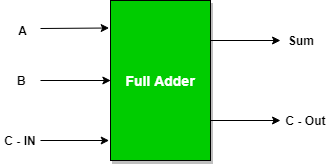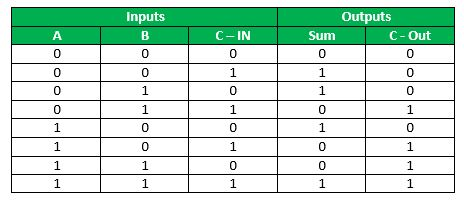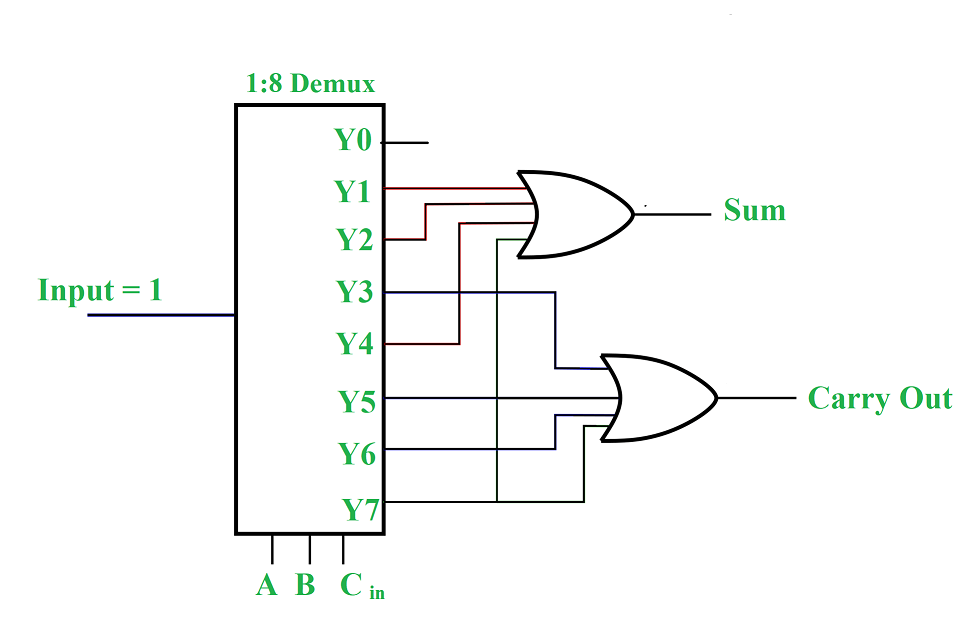Open in App
Not now

• Difficulty Level : Hard
• Last Updated : 21 Aug, 2022

Full Adder is a combinatorial circuit that computes the sum and carries out two input bits and an input carry. So it has three inputs – the two bits A and B, and the input carry Cin, and two outputs – sum S and output carry Cout.

Demultiplexer is a combinational circuit which has 1 input, n selection lines and generates 2n outputs. It is the reverse operation of multiplexer.

In this article, the Full Adder circuit is implemented by using the concept of the demultiplexer. By using the expression of the truth table of the Full Adder we connect a Demultiplexer with two OR gates and it performs an addition operation on three bits and produces output as sum and carry.### Truth Table of Full Adder:From the truth table, we can express Sum S and Carry out Cout as:

S(A, B, Cin)= (A’B’C) + (A’BC’) + (AB’C’) +(ABC)

Cout(A,B,Cin) = (A’BC) + (AB’C) + (ABC’) + (ABC)

which is,

S(A,B,Cin) = ∑(1,2,4,7)

Cout(A,B,Cin) = ∑(3,5,6,7)

Now, we will implement these SOP expressions using a demultiplexer.

### Implementation:

Steps to implement a Boolean function in a multiplexer:

1. First, find the number of input variables. If it’s 3 variable functions, then we need a 1:8 multiplexer. If it’s an n variable function, we require a 1:2n multiplexer.
2. Put the variables as selector lines of the multiplexer. A 1:2n multiplexer will have n selector lines.
3. Now, from the truth table of the function, find the minterms and grab the corresponding output lines of the demultiplexer, and put them into an OR gate. This makes sure that whenever any minterm of the function is high, the output is high.